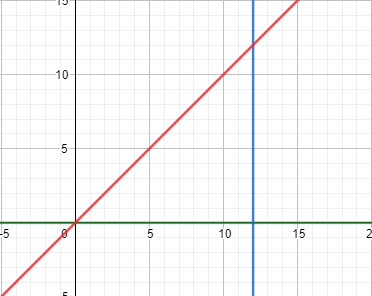# What is the area of the triangle enclosed by y = 0, x = 12 and y = x?

## Question:

What is the area of the triangle enclosed by {eq}y = 0, x = 12 {/eq} and {eq}y = x {/eq}?

## Area Enclosed by Functions

Suppose that we are interested in finding the area enclosed between two functions in a coordinate plane {eq}f(x) {/eq} and {eq}g(x) {/eq}, where {eq}f(x) {/eq} is our upper function and {eq}g(x) {/eq} is our lower function. In order to obtain the area between these two functions, the limits of integration {eq}x_1 {/eq} and {eq}x_2 {/eq} should be obtained which correspond to the intersection points of {eq}f(x) = g(x) {/eq}. Calculating the integral {eq}\displaystyle{\int_{x_1}^{x_2} (f(x)-g(x))~dx} {/eq} then allows us to obtain the total area.

Let's plot the three given functions:

{eq}y = 0\\ x = 12\\ y = x {/eq}

The plot is given below:Notice that our upper function is {eq}f(x) = x {/eq} and our lower function is {eq}g(x) = 0 {/eq}, as well as the limits of integration are {eq}x_1 = 0 {/eq} and {eq}x_2 = 12 {/eq}.

Therefore, to calculate the area of this triangle, we use the integral described:

{eq}A = \displaystyle{\int_{x_1}^{x_2} (f(x)-g(x))~dx = \int_{0}^{12} (x - 0)~dx = \frac{x^2}{2}|\begin{align*} &12\\ &0 \end{align*} =\frac{1}{2}\cdot 12^2 = 72} {/eq}

Therefore, the area of the triangle enclosed by the given curves is {eq}\boxed{A = 72} {/eq}